## Screenshots

Click the small images to view a full-size screen shot.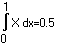Editor With the editor there is not only the standard functionality (saving, printing, printpreview, font, table, paragraph), but also the ability of integation and easy functions.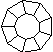Plot Function Plot functions f(x)Polar Plot Example for polar diagramBest fit Statistics: best fit, fast fourier transformation, histogram, smooth,....3D-Data Plot a function with 2 variables in a 3D-View and rotate. The rotation can be achieved by mouse interaction. It can be viewed from any direction, can be zoomed and panned.Optionally, axes and/or a bounding box may be displayed.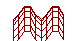Plot f(x,y) 3 dimensional GridnetVector For vectors basic operations (+-*/), crossproduct, spar product, unit vector and angle between two vectors can be evaluated. The professional version has more dimensions and a random generator.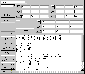Matrices For matrices basic operations (+-*/), inverse, determinante, rotation matrix and multiplication with a vector can be evaluated. The professional version can also split in 2 diagonal matrices, sum the diagonal elements, transpose the matrix, has more dimensions and a random generator.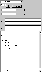2 Points For objects like point, line, plane and sphere distances, intersections, volume, area of squeres, area of a triangle can be calculated. In this example the distance of 2 Points is calculated.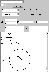Line - Circle In this example the intersection between a circle and a line is calculated.Plane - Line The combination of a line and a plane implies, that the line is in the plane, or intersects in a point, or they dont intersect. This example shows the intersection of line and a plane.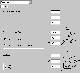Coordinates Cartesian coordinates, spherical coordinates und cylindrical coordinates can be transformed into each other.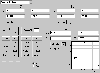Complex numbers Calculating with complex numbers. Elementar and trigonometric functions.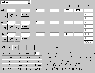Quaternions Calculating with quaternions. Elementar and trigonometric functions.Regular Polygon Paint regular polygons with inner circle, outer circle, radius, diagonals. Parameters like area, perimeter and the angles in center and corner are calculated. Because the number of sides is variable, this is good for explaining the area and perimeter of a circle.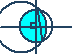Circles Circle: Sector, Segment, Intersection of 2 circles.